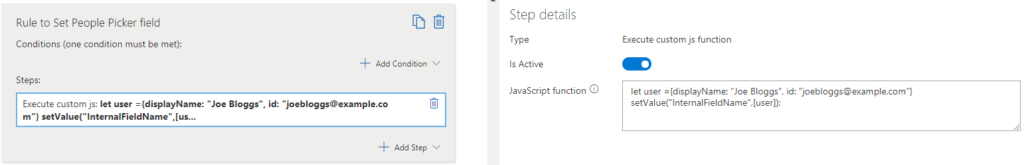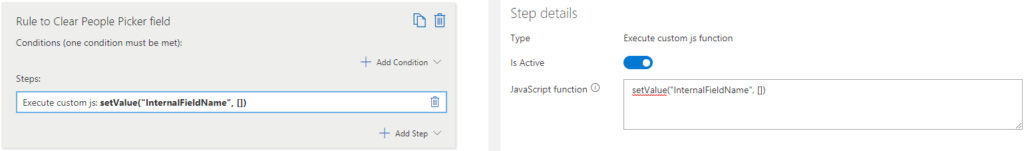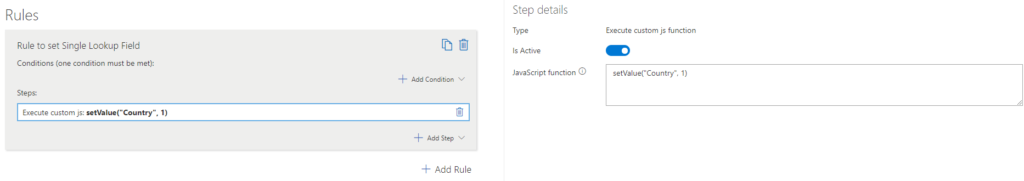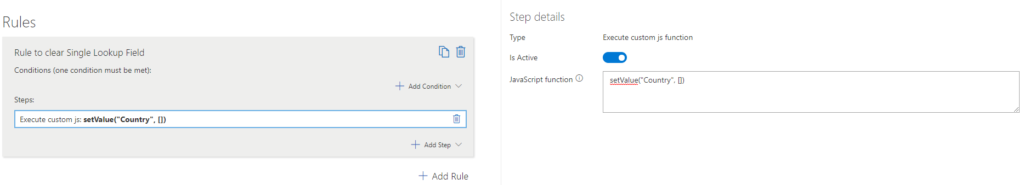How Can We Help?

# Set & clear field values using rules

You are here:

The setValue function within our JavaScript API allows you to set or clear any value on a form field. This function can be used within the Sintel Forms Designer Logic->Rules->Steps.

Below you will find code snippets for setting and clearing a field value for different field types.

#### User (both single and multi select)

Set it…

``````let user ={displayName: "Joe Bloggs", id: "joebloggs@example.com"}
setValue("InternalFieldName",[user]);
``````

Clear it…

``````setValue("InternalFieldName", [])
``````#### Lookup (single)

Set it…

``````setValue("InternalFieldName",ID of the lookup item)
``````

Clear it…

``````setValue("InternalFieldName", [])
``````#### Lookup (multi)

``````setValue("FieldName", [])
``````

#### Date (with and without the time part)

``````setValue("FieldName", null)
``````

#### Choice (single)

``````setValue("FieldName", null)
``````

#### Choice (multi)

``````setValue("FieldName", [])
``````

#### URL/image

``````setValue("FieldName", null)
setValue("Url", {description: "Test", url: "https://www.test.com"})
``````

#### Currency/number

``````setValue("FieldName", "")
``````

#### Text

``````setValue("FieldName", "")
``````